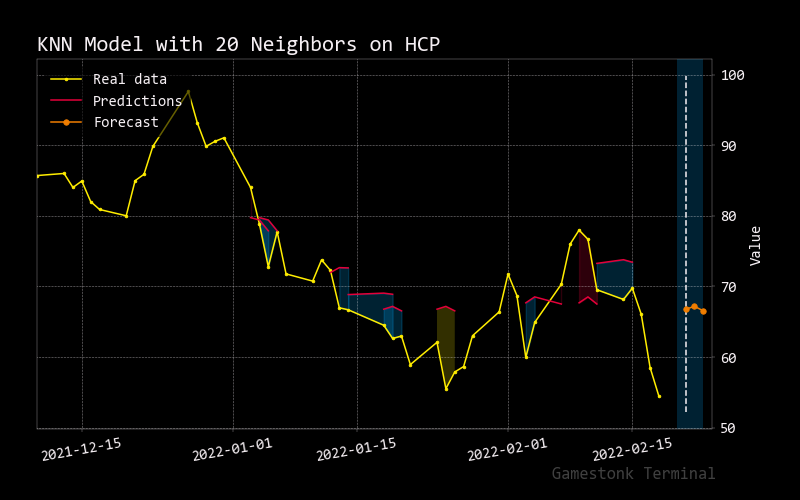# Knn

``````usage: knn [-i N_INPUTS] [-d N_DAYS] [-j N_JUMPS] [-n N_NEIGHBORS] [-e S_END_DATE] [-t VALID_SPLIT] [-p {normalization,standardization,minmax,none}] [--no_shuffle] [-h]
``````

K nearest neighbors is a simple algorithm that stores all available cases and predict the numerical target based on a similarity measure (e.g. distance functions).

``````optional arguments:
-i N_INPUTS, --input N_INPUTS
number of days to use as input for prediction.
-d N_DAYS, --days N_DAYS
prediction days.
-j N_JUMPS, --jumps N_JUMPS
number of jumps in training data.
-n N_NEIGHBORS, --neighbors N_NEIGHBORS
number of neighbors to use on the algorithm.
-e S_END_DATE, --end S_END_DATE
The end date (format YYYY-MM-DD) to select for testing
-t VALID_SPLIT, --test_size VALID_SPLIT
Percentage of data to validate in sample
-p {normalization,standardization,minmax,none}, --pp {normalization,standardization,minmax,none}
pre-processing data.
--no_shuffle          Specify if shuffling validation inputs.
-h, --help            show this help message
``````